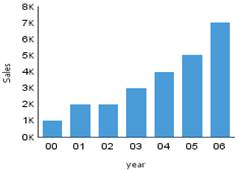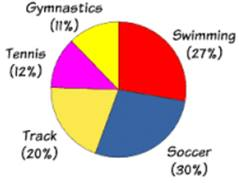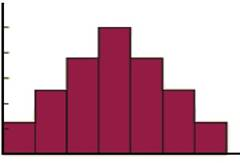# 8th Class Chapter No 15 - Introduction to Graphs in Maths for CBSE NCERT

• Graphical presentation of data is easier to understand.
(i) A bar graph  is used to show comparison among categories.
(ii) A pie graph is used to compare parts of a whole.
(iii) A Histogram is a bar graph that shows data in intervals.
• A  line graph displays data that changes continuously over periods of time.
• A line graph which is a whole unbroken line is called a  linear graph.
• For fixing a point on the graph sheet we need, x-coordinate and y-coordinate.
• The relation between dependent variable and  independent variable is shown through a graph.
• A Bar Graph: A pictorial representation of numerical data in the form of bars (rectangles) of uniform width with equal spacing. The length (or height) of each bar represents the given number.• A Pie Graph: A pie graph is used to compare parts of a whole. The various observations or components are represented by the sectors of the circle.• A Histogram: Histogram is a type of bar diagram, where the class intervals are shown on the horizontal axis and the heights of the bars (rectangles) show the frequency of the class interval, but there is no gap between the bars as there is no gap between the class intervals.• Linear Graph: A line graph in which all the line segments form a part of a single line.
• Coordinates: A point in Cartesian plane is represented by an ordered pair of numbers.
• Ordered Pair: A pair of numbers written in specified order.
Posted in 8th on February 13 2019 at 03:29 PM

### CBSE NCERT 8th CLASS Maths OTHER CHAPTERS